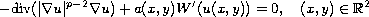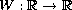Electron. J. Diff. Equ., Vol. 2010(2010), No. 15, pp. 1-10.

### Entire solutions for a class of p-Laplace equations in R^2 Zheng Zhou

Abstract:
We study the entire solutions of the p-Laplace equationwhere a(x,y) is a periodic in x and y, positive function. Hereis a two well potential. Via variational methods, we show that there is layered solution which is heteroclinic in x and periodic in y direction.

Submitted September 15, 2009. Published January 21, 2010.
Math Subject Classifications: 35J60, 35B05, 35B40.
Key Words: Entire solution; p-Laplace Allen-Cahn equation; Variational methods.

Show me the PDF file (230 KB), TEX file, and other files for this article.Zheng Zhou College of Mathematics and Econometrics Hunan University, Changsha, China email: zzzzhhhoou@yahoo.com.cn